# 有源带通滤波器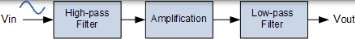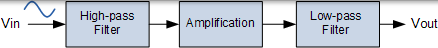### 有源带通滤波器电路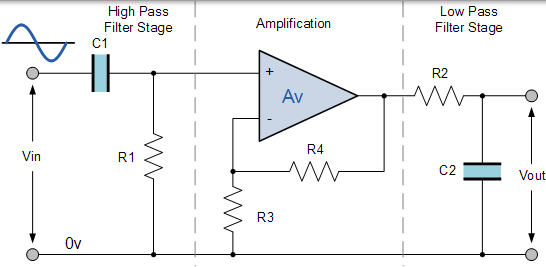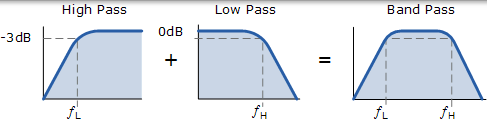### 有源带通频率响应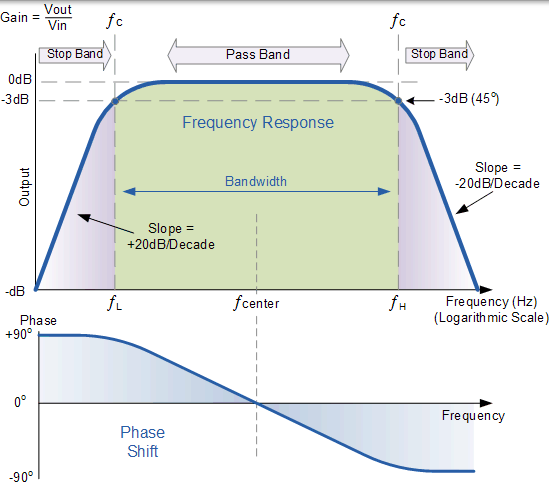### 反相带通滤波器电路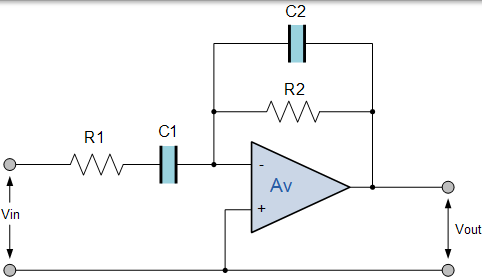$$\text { Voltage Gain } = - \frac { R _ { 2 } } { R _ { 1 } } , \quad f _ { C _ { 1 } } = \frac { 1 } { 2 \pi R _ { 1 } C _ { 1 } } , f c _ { 2 } = \frac { 1 } { 2 \pi R _ { 2 } C _ { 2 } }$$

## 多反馈带通有源滤波器

### 无限增益多反馈有源滤波器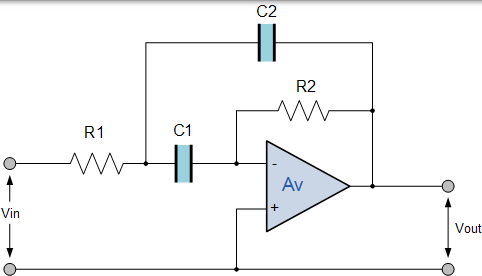$$f _ { \mathrm { r } } = \frac { 1 } { 2 \pi \sqrt { \mathrm { R } _ { 1 } \mathrm { R } _ { 2 } \mathrm { C } _ { 1 } \mathrm { C } _ { 2 } } } \quad \mathrm { Q } _ { \mathrm { BP } } = \frac { f _ { \mathrm { r } } } { \mathrm { BW } _ { ( 3 \mathrm { d } \mathrm { B } ) } } = \frac { 1 } { 2 } \sqrt { \frac { \mathrm { R } _ { 2 } } { \mathrm { R } _ { 1 } } }$$

## 有源带通滤波器示例 No1

$$\mathrm { Av } = 1 = - 2 \mathrm { Q } ^ { 2 } \quad \therefore \mathrm { Q } _ { \mathrm { BP } } = \sqrt { \frac { 1 } { 2 } } = 0.7071 \\ Q = 0.7071 = \frac { 1 } { 2 } \sqrt { \frac { R _ { 2 } } { R _ { 1 } } } \quad \therefore \frac { R _ { 2 } } { R _ { 1 } } = \left( \frac { 0.7071 } { \frac { 1 } { 2 } } \right) ^ { 2 } = 2$$

$$\begin{array} { c } { f _ { \mathrm { r } } = 1,000 \mathrm { Hz } = \frac { 1 } { 2 \pi \mathrm { C } \sqrt { \mathrm { R } _ { 1 } \mathrm { R } _ { 2 } } } } \ { \therefore \mathrm { C } = \frac { 1 } { 2 \pi f _ { \mathrm { r } } \sqrt { \mathrm { R } _ { 1 } \mathrm { R } _ { 2 } } } = \frac { 1 } { 2 \pi 1000 \sqrt { 10,000 \times 20,000 } } = 11.2 \mathrm { nF } } \end{array}$$

## 谐振频率点

$$f_{r} = \sqrt { f _ { L } \times f _ { H } }$$

• ƒR 是谐振或中心频率
• ƒL 是 -3dB 的下截止频率
• ƒH 是 -3db 的上截止频率

$$f r = \sqrt { 200 \times 600 } = \sqrt { 120,000 } = 346 \textrm{ Hz}$$

## Q-Factor 或品质因数

$$\alpha = \frac { 1 } { Q }$$

$$\xi = \frac { \alpha } { 2 }$$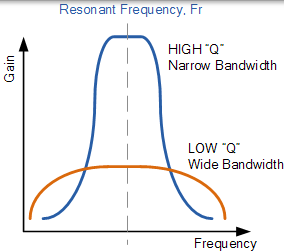$$Q = \frac { \text { Resonant Frequency } } { \text { Bandwidth } }$$

346Hz / 400Hz = 0.865。请注意，Q 是比率，没有单位。